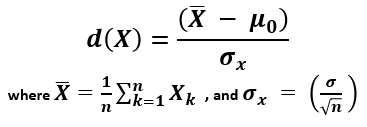# P-value to Z-score Calculator

Use this P to Z calculator to easily convert P-values to Z-scores (standard scores) and see if a result is statistically significant. Supports one-tailed and two-tailed p-values. Detailed information about what a Z-score is.

Share calculator:

Embed this tool:
get code

## Using the p-value to z-score calculator

If you have a p-value statistic for a given set of data and want to convert it to its corresponding Z score this P to Z calculator will help you accomplish that. Simply enter the P-value and choose whether it was computed for a one-tailed or two-tailed significance test to calculate the corresponding Z score using the inverse normal cumulative PDF (probability density function of the normal distribution). If you have made a directional inference, saying something about the sign or direction of the effect, then your p-value should have been calculated as one-tailed, corresponding to a one-sided composite null hypothesis. If the direction of the effect did not matter in the initial p-value calculation, select two-tailed, which corresponds to a point null hypothesis.

Since the normal distribution is symmetrical, it does not matter if you are computing a left-tailed or right-tailed p-value: just select one-tailed and you will get the correct result for the direction in which the observed effect is. If you want the Z score for the other tail of the distribution, just reverse its sign, e.g. 1.7 becomes -1.7.

## What is a "Z score"

The Z-score is a statistic showing how many standard deviations away from the normal, usually the mean, a given observation is. It is often called just a standard score, z-value, normal score, and standardized variable.

The standard score is calculated by estimating the variance and standard deviation, then deriving the standard error of the mean, after which a standard score is calculated using the formula :X (read "X bar") is the arithmetic mean of the population baseline or the control, μ0 is the observed mean / treatment group mean, while σx is the standard error of the mean (SEM, or standard deviation of the error of the mean).

The interpretation of a Z-score has problems similar to that of p-values and confidence intervals, on which you can read more in our respective pages.

One of the applications of standard scores is in constructing prediction intervals. A prediction interval [L,U] is an interval such that a future observation X will lie in the interval with a given probability, i.e. 90%.

## P value to Z-score conversion table

Below are some commonly encountered p-values and their corresponding standard scores, assuming a one-tailed hypothesis.

Percentile and p-value to standard score table
PercentileP-valueStandard score (Z)
80% 0.2000 0.8416
90% 0.1000 1.2816
95% 0.0500 1.6449
97.5% 0.0250 1.9600
98% 0.0200 2.0537
99% 0.0100 2.3263
99.9% 0.0010 3.0902
99.95% 0.0005 3.2905